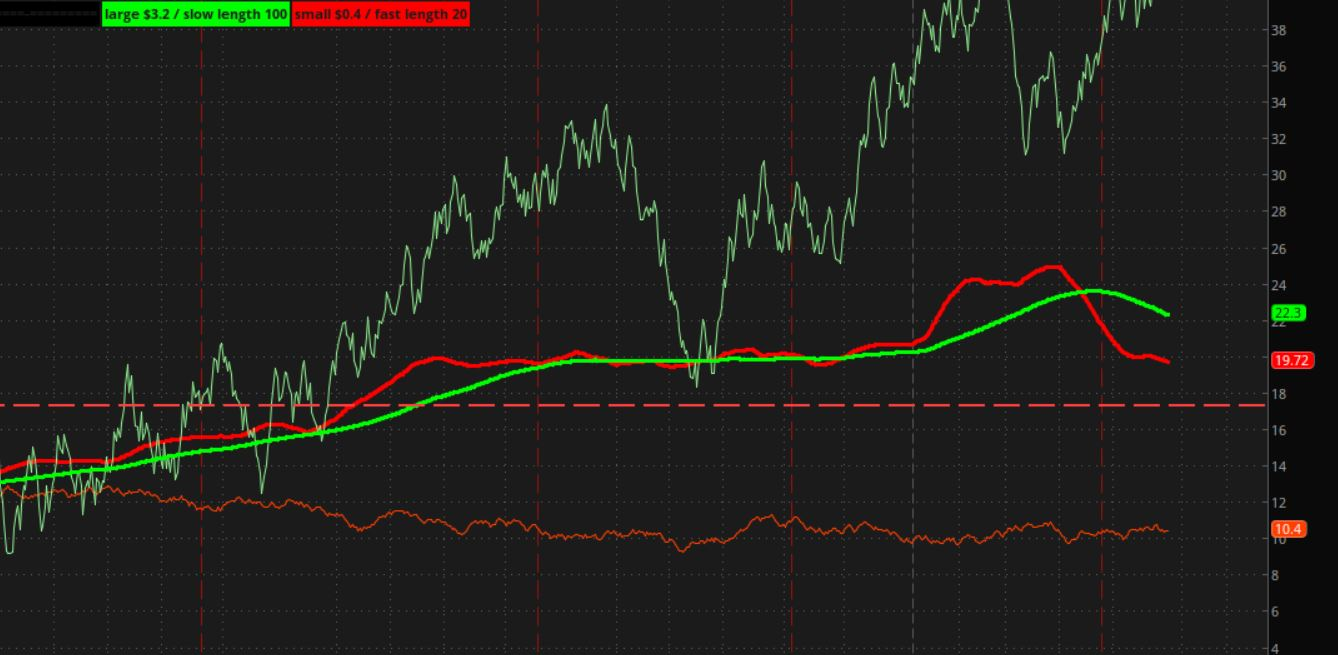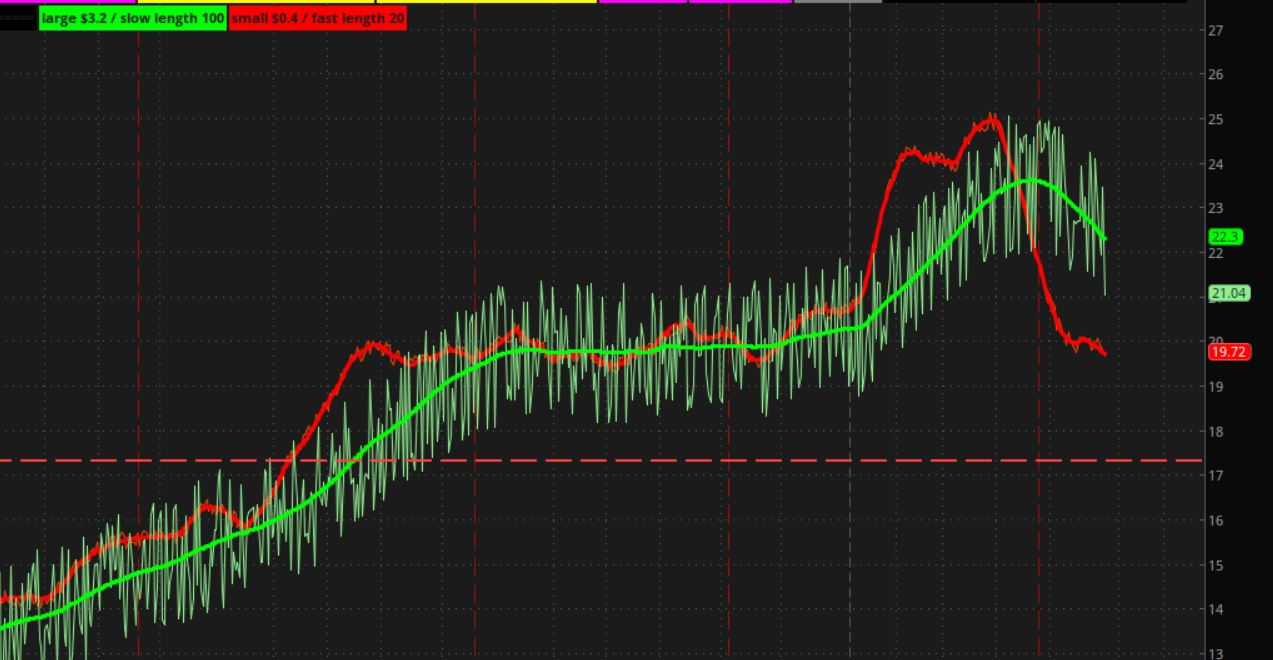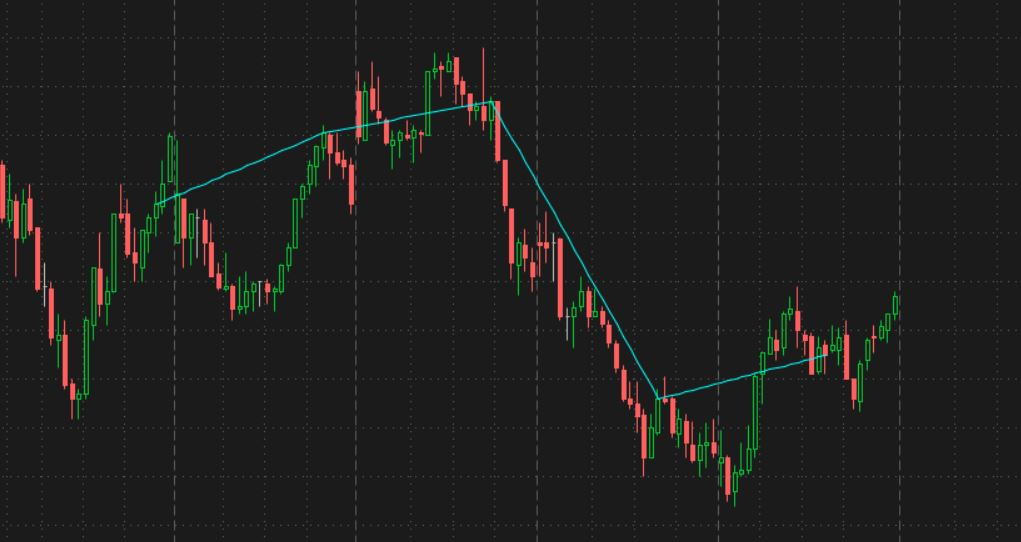# Random() function

#### DanielManahan

##### Member
I want to create a fake stock chart using the random() function

Random returns a value between 0 to 1 which can be multiplied and manipulated with other math operators.

but what I am not seeing how to do is get a fractal pattern noise of larger random mixed with small random patterns because both the large and small share the same aggregation periods.

I need a large random pattern on a slow moving random pattern and a small random pattern on a fast moving pattern to create the fractal.

how do do this?

the manual is not clear what can be put between the () part of the Random() function. I experimented with putting number and/or stock symbols in the () but just get an error.

how to create a fractal using Random() so the results are something that looks and behaves like a real stock chart?

if this can't be done, then that proves that stocks are not random, and are not fractals.

I want to create a fake stock chart using the random() function

Random returns a value between 0 to 1 which can be multiplied and manipulated with other math operators.

but what I am not seeing how to do is get a fractal pattern noise of larger random mixed with small random patterns because both the large and small share the same aggregation periods.

I need a large random pattern on a slow moving random pattern and a small random pattern on a fast moving pattern to create the fractal.

how do do this?

the manual is not clear what can be put between the () part of the Random() function. I experimented with putting number and/or stock symbols in the () but just get an error.

how to create a fractal using Random() so the results are something that looks and behaves like a real stock chart?

if this can't be done, then that proves that stocks are not random, and are not fractals.

this may help. this is a test study, that finds random numbers with 2 slightly different methods. they are basically the same, they just use different inputs.

the first section asks for 2 numbers, the limits of a range, to find random numbers, including and within.
it draws 20 labels with numbers

the second method asks for a quantity of numbers and a starting number
it draws 10 labels with numbers and bubbles under the bars

Ruby:
``````# random_numbers_labels_00

# random numbers.

#  --- method 1
# pick a range of numbers for random values
input highest_number = 6;
input lowest_number = -2;

def rng = (highest_number - lowest_number + 1);
def rand = floor(lowest_number +  (random() * rng));

addlabel(1, "random numbers, " + lowest_number + " to " + highest_number , color.cyan);

addlabel(1, " >>> " , color.cyan);

addlabel(1, " <<< " , color.cyan);

# ------------------------------------

#  --- method 2

input spacer = no;

#DefineGlobalColor("color2", CreateColor(128, 0, 128));
DefineGlobalColor("color2", color.light_gray);
# put this code in for a color,  GlobalColor("color2")

# qty of colors
input number_qty = 10;
input start_number = 1;
def end = start_number + number_qty -1;

addlabel(1, number_qty + " random numbers, starting at " + start_number , color.cyan);

# create random numbers, 1 to 10
def c = floor(random() * number_qty) + start_number;

#``````

this may help. this is a test study, that finds random numbers with 2 slightly different methods. they are basically the same, they just use different inputs.

the first section asks for 2 numbers, the limits of a range, to find random numbers, including and within.
it draws 20 labels with numbers

the second method asks for a quantity of numbers and a starting number
it draws 10 labels with numbers and bubbles under the bars

Ruby:
``````# random_numbers_labels_00

# random numbers.

#  --- method 1
# pick a range of numbers for random values
input highest_number = 6;
input lowest_number = -2;

def rng = (highest_number - lowest_number + 1);
def rand = floor(lowest_number +  (random() * rng));

addlabel(1, "random numbers, " + lowest_number + " to " + highest_number , color.cyan);

addlabel(1, " >>> " , color.cyan);

addlabel(1, " <<< " , color.cyan);

# ------------------------------------

#  --- method 2

input spacer = no;

#DefineGlobalColor("color2", CreateColor(128, 0, 128));
DefineGlobalColor("color2", color.light_gray);
# put this code in for a color,  GlobalColor("color2")

# qty of colors
input number_qty = 10;
input start_number = 1;
def end = start_number + number_qty -1;

addlabel(1, number_qty + " random numbers, starting at " + start_number , color.cyan);

# create random numbers, 1 to 10
def c = floor(random() * number_qty) + start_number;

#``````
thanks I am looking to plot a line such that the line would look like the fractal chart pattern we see in a typical stock trading chart where there are small trends inside bigger trends inside bigger trends. plot a fractal line.

My code to try and get a slower and larger random pattern and use it with a faster and smaller random pattern by using the Aggregation periods 5 minute for the fast and 15 minute for the slow

but the syntax is not working

declare lower;

def agg05 = aggregationPeriod.five_MIN;
def agg15 = aggregationPeriod.FIFTEEN_MIN;

def fastNoise = (Random()(Period = Agg05))/2;
def slowNoise = (Random()(Period = Agg15))*5;

plot line = fastNoise + slowNoise;

My code to try and get a slower and larger random pattern and use it with a faster and smaller random pattern by using the Aggregation periods 5 minute for the fast and 15 minute for the slow

but the syntax is not working

declare lower;

def agg05 = aggregationPeriod.five_MIN;
def agg15 = aggregationPeriod.FIFTEEN_MIN;

def fastNoise = (Random()(Period = Agg05))/2;
def slowNoise = (Random()(Period = Agg15))*5;

plot line = fastNoise + slowNoise;
this is the second post in a day , where you are asking for,
faster and smaller random pattern....
these terms are too vague and make no sense at all.

can you use numbers and not use large, short, small, long, to descibe what you want to see?

agg desciptions times are in milliseconds. if you divide them by 60,000 you will have minutes.

agg variables are used in price variables like this,
def x = close( period = agg05 );

your formulas with period = , are not inside of a price variable, like close.

you can't put random() next to period =

i made a guess and started to make a study that combines a random number and an average with a long length, to plot a random, jittery line.

okay thanks for trying

it seems as though people don't understand what I want, so my original post is not appropriate and should be re asked in a way that is more clear.

thanks I am looking to plot a line such that the line would look like the fractal chart pattern we see in a typical stock trading chart where there are small trends inside bigger trends inside bigger trends. plot a fractal line.

i am still confused on what you are asking for.

i made this study to draw a couple of wavy lines. maybe it will help you describe what you are after, similar to these or not.

there are 2 sections

line set 1. green
. large random numbers, in the range of 0 to \$3.20
. an average with a long length of 100

line set 2. red
. small random numbers, in the range of 0 to \$0.40
. an average with a short length of 20

i picked those price and length numbers at random.

options:
can turn off the main candles, to make it easier to see just these lines.

can pick how random numbers are added,
1. to the "previous combined value"
. this ignores the average and adds a random number to the previous random summation. it starts with the average value at bar #1.

2. to the "average"
. this adds a random number to an average. these oscillations closely follow the average line.

Ruby:
``````# random_numbers_2signals_combined_00

# random numbers.
#   have a large random pattern on a slow moving random pattern
#   and a small random pattern on a fast moving pattern

# -----------------------------------------
def bn = BarNumber();
def na = Double.NaN;

# turn off main chart bars
input turn_off_main_candles = yes;
HidePricePlot(turn_off_main_candles);

input add_random_data_to = { default "previous combined value", "average" };

#---------------------------------------------
#  a large random pattern on a slow moving random pattern

input show_slow_signals_green = yes;

input large_random = 3.20;
input slow_len = 100;
input avg1_type =  AverageType.SIMPLE;
def ma1 = MovingAverage(avg1_type, close, slow_len);

def larg_rand = (Floor(Random() * large_random * 100)/100) - (large_random / 2);

plot z1 = if show_slow_signals_green then ma1 else na;
z1.SetDefaultColor(Color.green);
z1.setlineweight(3);

def z2b;
case "previous combined value":
z2b = if bn == 1 then z1 else if isnan(close) then na else if show_slow_signals_green then (z2b + larg_rand) else na;
case "average":
z2b = if show_slow_signals_green then (ma1 + larg_rand) else na;
}

#def z2a = if show_slow_signals_green then (ma1 + larg_rand) else na;
#def z2b = if bn == 1 then z1 else if show_slow_signals_green then (z2b + larg_rand) else na;

plot z2 = z2b;
z2.SetDefaultColor(Color.light_green);
z2.setlineweight(1);

addlabel(show_slow_signals_green, "large \$" + large_random + " / slow length " + slow_len, color.green);

#---------------------------------------------
#  a small random pattern on a fast moving pattern

input show_fast_signals_red = yes;

input small_random = 0.40;
input fast_len = 20;
input avg2_type =  AverageType.SIMPLE;
def ma2 = MovingAverage(avg2_type, close, fast_len);

def small_rand = (Floor(Random() * small_random * 100)/100) - (small_random / 2);

plot z3 = if show_fast_signals_red then ma2 else na;
z3.SetDefaultColor(Color.red);
z3.setlineweight(3);

def z4b;
case "previous combined value":
z4b = if bn == 1 then z3 else if isnan(close) then na else if show_fast_signals_red then (z4b + small_rand) else na;
case "average":
z4b = if show_fast_signals_red then (ma2 + small_rand) else na;
}

plot z4 = z4b;
z4.SetDefaultColor(Color.light_red);
z4.setlineweight(1);

addlabel(show_fast_signals_red, "small \$" + small_random + " / fast length " + fast_len, color.red);
#``````

F 1hr random # added the prev valueF 1hr random # added to an averageokay thanks for trying

it seems as though people don't understand what I want, so my original post is not appropriate and should be re asked in a way that is more clear.
sometimes people have trouble getting an idea out of their head. keep trying to explain it or find visual examples that might be close.
and just because i don't get it, doesn't mean that someone won't show up tomorrow who understands it.

Last edited:
I will study your code to see if that is what I am looking for.

the idea is actual as simple as it sounds. I want to create a stock pattern.

stocks move in random movements as nobody can predict the next move

they are random on the year, random on the month, random on the week, random on the day, random on the half hour, and random on the minute. they are always random and never predictable.

each of those different random periods: year, month, week, day, half hour, minute will have its own random pattern and unrelated to each other pattern. they will be random patterns that exist inside each other.

like a fractal

so I started with the Random() function

the problem I had was that it used the current aggregation of the chart, so the up and down that Random() returns a value from zero to one was happening on each minute which is good but only for the minute and didn't represent the year, month, week, day, half hour.

I will check out your code because the Z2 line in light green looks the closest to what I want.

looked at your code and the one that looks like what I want Z2 is relying on the stock chart CLOSE rather than creating its own pattern.

I suppose this could work if I normalize the stock chart with Close - Close to get a price line of zero, flat, and then work from that. the idea is to use the aggregations of the chart which is normalized to zero so that I can get multiple random patterns on different time frames borrowed from piggy backing on the zero line close points.

thanks I will try to come up with something and re ask the question if and when I come to a problem.

okay the problem I am having is I can't see the aggregation of a higher time frame within a lower time frame.

if I am in the one minute chart I can't see the data for the 1 month using AggregationPeriod.month doesn't work.

is there any work around for this?

okay the problem I am having is I can't see the aggregation of a higher time frame within a lower time frame.

if I am in the one minute chart I can't see the data for the 1 month using AggregationPeriod.month doesn't work.

is there any work around for this?
1 minute to a month is a huge difference. your 1 minute setting will need to be at least 30 days.
a 1 minute 1 day chart setting isn't going to have enough history data to show.

here is a test study to experiment with
Code:
``````# read close from another timeframe (agg)
input agg = AggregationPeriod.day;

# example , get price from an agg time
def test1 = close(period = agg);

plot z = test1;
z.setdefaultcolor(color.cyan);
#``````

thank you, this is what I feared would happen.

it is stair stepping the line which isn't doing what I expected, it is doing what the limitations of the scripting language allows

what is expected is a diagonal line from data point to data point

I am still learning the limitations of the software and limitations of the language.

sharing a public image from my google drive image

Yellow line is expected, cyan line is the results of the code and limitations of the software

Last edited:
•MerryDay
thank you, this is what I feared would happen.

it is stair stepping the line which isn't doing what I expected, it is doing what the limitations of the scripting language allows

what is expected is a diagonal line from data point to data point

I am still learning the limitations of the software and limitations of the language.

sharing a public image from my google drive image

Yellow line is expected, cyan line is the results of the code and limitations of the software

if you want diagonal lines, between 2 points, that aren't on side by side candles, there are a couple ways to do that.

the easiest is to use approximation with plot.
find a way to set the desired values, from the desired bars, into a variable.
for the other values, set the variable equal to double.nan.
then plot the variable, and use EnableApproximation().
. example, price levels exist only in a variable, on every 20th bar.

another way is to use a fold loop to look ahead and find the next data point.
then calculate the slope af a line between the 2 points, and add the y increment on each bar.

Code:
``````# test_plot_approx_skipbn

# test plotting using approx
# create test data and try to plot diangonal lines between them
# set the close to a var , every x bars

def bn = barnumber();
def na = double.nan;

input skipbars = 24;

def en = if (bn % skipbars) == 0 then 1 else 0;

def data = if en then close else na;

plot z = data;
Z.EnableApproximation();
z.setdefaultcolor(color.cyan);
#``````

https://tlc.thinkorswim.com/center/reference/thinkScript/Functions/Look---Feel/EnableApproximation@halcyonguy

here is my code

this is not expected. I need to combine both lines the larger (input 390 for minutes in the day) with (input 30 for half hour) and I expect the smaller fractal to go up and down based on additive math with the larger fractal

the end result will be to mimic a stock chart

declare lower;

def bn = barnumber();
def na = double.nan;
def zeroPlot = close-close; #Normalize the current stock chart to a zero line

def skipbarsDay = 390;
def skipbarsHalfHour = 30;
#def skipbarsFiveMin = 5;
#def skipbarsMin = 1;

def dayFractal = if (bn % skipbarsDay) == 0 then 1 else 0;
def fractalDay = if dayFractal then zeroPlot else na;

def HalfHourFractal = if (bn % skipbarsHalfHour) == 0 then 1 else 0;
def fractalHalfHour = if HalfHourFractal then zeroPlot else na;

def w = fractalDay+Random();
def x = fractalHalfHour+Random();
plot wFractal = w;
plot xFractal = x;
plot combinedFractal = w+x;

wFractal.EnableApproximation();
xFractal.EnableApproximation();
combinedFractal.EnableApproximation();

wFractal.setdefaultcolor(color.cyan);
xFractal.setdefaultcolor(color.pink);
combinedFractal.setdefaultcolor(color.light_Green);

I expected the cyan and pink line to combine and create the green line I drew in. instead it creates the light green line, not expected

### Not the exact question you're looking for?

87k+ Posts
185 Online## The Market Trading Game Changer

Join 2,500+ subscribers inside the useThinkScript VIP Membership Club
• Exclusive indicators
• Proven strategies & setups
• Private Discord community
• Exclusive members-only content
• 1 full year of unlimited support

What is useThinkScript?

useThinkScript is the #1 community of stock market investors using indicators and other tools to power their trading strategies. Traders of all skill levels use our forums to learn about scripting and indicators, help each other, and discover new ways to gain an edge in the markets.

How do I get started?

We get it. Our forum can be intimidating, if not overwhelming. With thousands of topics, tens of thousands of posts, our community has created an incredibly deep knowledge base for stock traders. No one can ever exhaust every resource provided on our site.

If you are new, or just looking for guidance, here are some helpful links to get you started.

What are the benefits of VIP Membership?